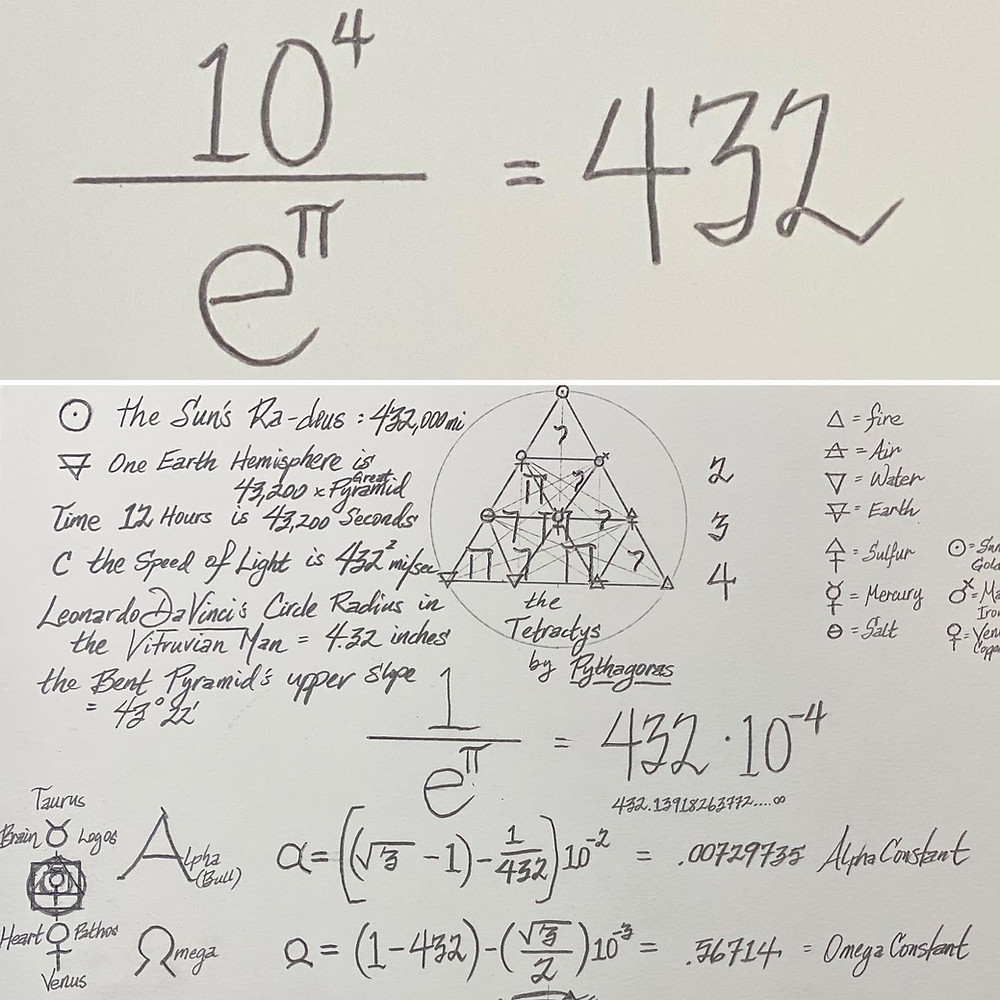Search
• Jamie

# 1/(e^π) = 432(10^-4)

NEW DISCOVERY TODAY (10/20/2020): 1/(e^π) = 432(10^-4). A few months ago I found that the physics constant Alpha (α) could be derived (something the late and great Nobel Laureate Richard Feynman PhD predicted would never happen) to seven decimal digits of accuracy using the following equation: α (.00729735) = (((3^.5)-1)-1/432)10^-2. On the same day, I realized that the Omega constant (0.56714) could also be achieved using a strangely similar equation (by simply rearranging the same numbers and interchanging exponents with 2 and 3): Ω =((1-432)-(3^.5)/2))10^-3. Again another way to derive Ω is also approx = 1-.432..... Today, while working to unravel (e^iπ)+1 = 0; I found that the reciprocal of e^π = 432....Let's consider the following "coincidences": 1. The Sun's Radius is approx 432,000 miles (Jupiter's Radius is likewise approx 43,200 miles); 2. One Earth Hemisphere is 43,200:1 (versus the Great Pyramid); 3. There are 43,200 seconds of "Daytime = 12 Hours"; 4. 432^2 is the closest whole number that squares to the Speed of Light; 5. The Bent Pyramid's upper slope is 43°22'; 6. Leonardo's (Da Vinci) Vitruvian Man Circle at the original scale = 4.32 inches. 7. The Pythagorean Tetraktys and Just Tuning is based on 432/Hz. 8. A circle with Circumference of 432 units possesses a Diameter of 137.509 units (The Golden Angle). The number 432 is so prevalent in esoteric Rosicruscian/Hermetic reference and is found at the very epicenter of both Mathematical and Physical Constants......with the CENTER of the (432) Tetraktys signifying THOTH and at the same time, often depicted with the name YHWH, ONE has to at least consider the possibility of a connection.....